Python is a great programming language for data analysis. With tons of packages for data handling it is the best choice for data analysts , algorithm developers and more

Lets get started with understanding the relationship between some very popular data packages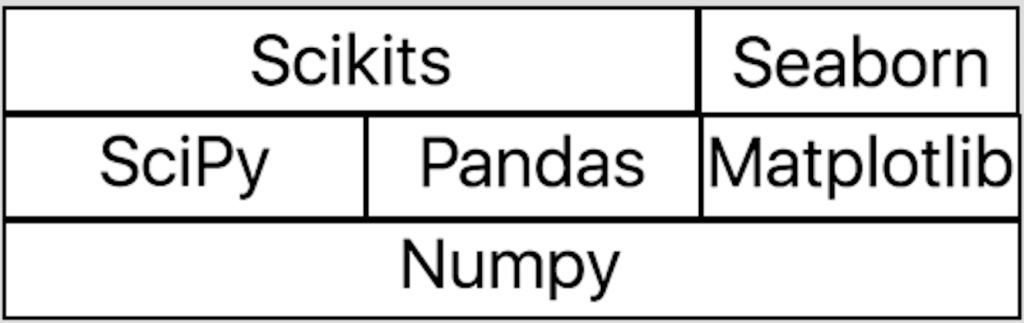Numpy is package for multi dimension arrays – very effective implementation

Scipy – package for scientific programming , mathematics , signal processing and more

Pandas – package for data handling

Matplotlib – package for data visualization (graphs)

Seaborn – extend Matplotlib with statistical graphs

Scikits – many extensions to spicy for specific fields like x-ray, image processing , deep learning and many more

### Scikit Learn

Scikit Learn is one of the scikit packages and its a very easy to use Machine learning package. It implements many machine learning algorithms and all you need to know is which algorithm solves your problem. Machine learning is a statical field , the algorithm learn from data provided and helps you predict the result of data not provided

To understand machine learning and how can we use it lets see simple example:

First we need to import all the required packages:

```import numpy as np
import sklearn.linear_model as skl
import pylab as py
import pandas as pd
import seaborn as sb```

We will use linear regression model. It is the easiest algorithm to start with – you have a function f(x)=y , you have some pairs of (x,y) that match the function and you want to predict y for other x values. This can work also for more than one dimension for example you have f(x,y,z) = w and you want to predict w for new tuples of (x,y,z)

To create a linear regression model:

`model = skl.LinearRegression()`

Each algorithm in scikit-learn has a fit function to train the model and a predict function to predict output for new values

Lets create some data:

```xval = np.array([1,2,3,4,5]).reshape(-1,1)
yval = [1,2,3,4,5]```

we tell the model that f(1) = 1 , f(2) = 2 , f(3) = 3, and so on – very trivial

Lets train the model:

`model.fit(xval,yval)`

Now lets predict y for new data :

```>>> model.predict(12)
array([ 12.])
>>> model.predict(44
array([ 44])```

we can see that its 100% correct because its very trivial. Lets add some errors:

```xval = np.array([1,2,3,3,4,3,6,8,9,10]).reshape(-1,1)
yval = [1,2,3,4,5,6,7,7,9,10]
model.fit(xval,yval)```

We can use matplotlib to visualize the data:

`py.scatter(xval,yval)`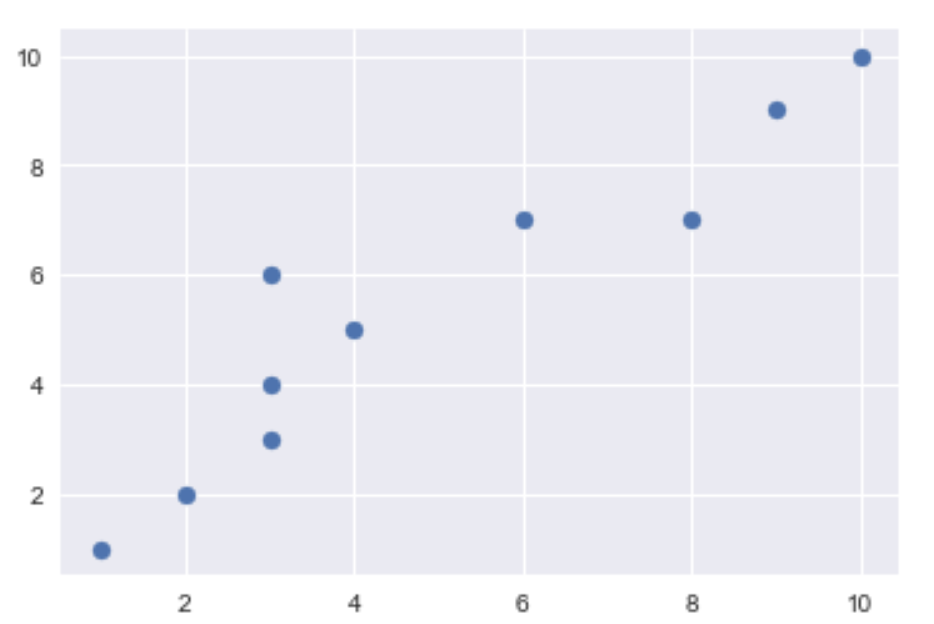We can see from the graph that there is linear correlation between x and y but it has some errors:

lets try to predict values:

```>>> model.predict(12)
array([ 11.66141732])
>>> model.predict(44
array([ 39.88188976])```

We can see that the results has errors but it is close to the real results

#### More Dimensions

Real world problems have more than one dimension for example: given a height, weight and shoes size – tell me if its a male of female.

Lets make some data with more dimensions:

```samp=np.array([[1,2,300,14],
[9,3,1,95],
[5,7,11,58],
[4,8,14,57],
[2,1,2,27],
[9,9,7,100],
[12,3,21,126],
[29,12,3,309],
[2,40,11,90],
[21,32,4,270],
[7,13,8,79],
[17,2,19,172],
[13,24,13,159]])
df=pd.DataFrame(samp, columns=['X','Y','Z','W'])```

To handle multiple dimensions we use Pandas dataframe: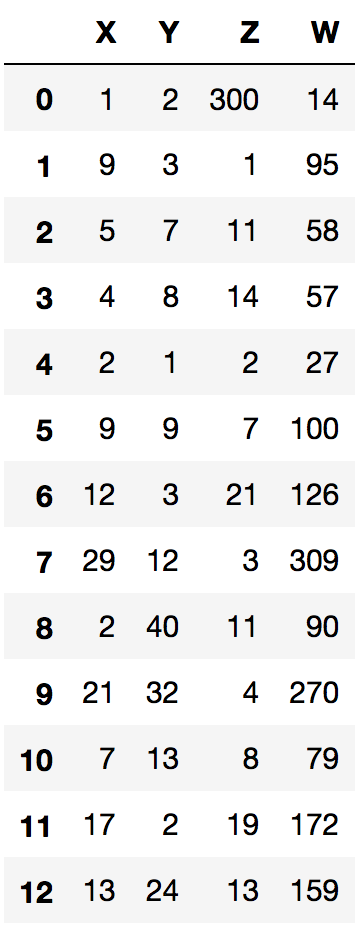We now can use seaborn pairplot graph to find relations between columns:

`sb.pairplot(df)`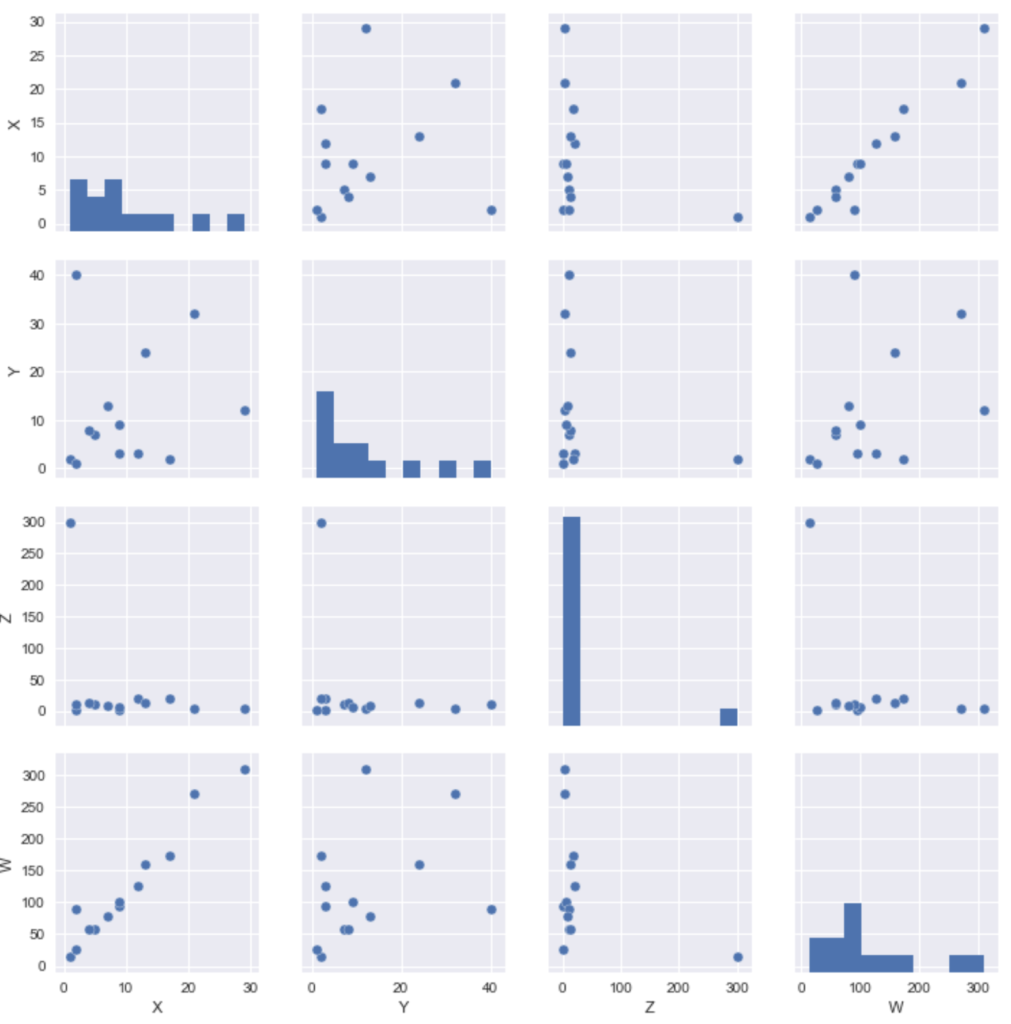We can see the relations between the variables for example we can see that x and w has strong relation

### Splitting the data – train and test

When we build a model, we want to check its correctness , to do that we split the data we have to train data and test data. We use the train data to fit the model and then check with our test data to see if the model predicts the results we have. For example we have above 13 rows. we take only 8 of our model:

```xval=df[:8][['X','Y','Z']] # only first 8 rows
yval=df[:8][['W']]
model.fit(xval,yval)```

Now lets take the other 5 rows and check the values the model predicts:

```>>> model.predict(df[8:][['X','Y','Z']])
array([[  68.12886489],
[ 249.65152055],
[  87.91908068],
[ 175.75498023],
[ 160.41262073]])```

Now compare it with the real values we know: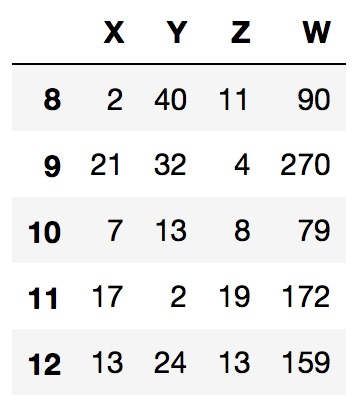As you can see rows 8+9 are not so close to the real values but 10-12 much more.

Note that for learning purpose we are using very small amount of data , for real world scenarios we need much more than 13 records.

We can change the model in some ways: Use different algorithm or use part of the variable for example:

Lets check the model coefficient

```>>> model.coef_
array([[  1.00253720e+01,   1.12411075e+00,  -4.78200390e-03]])```

We can see that z has small impact (0.004) so it is good idea to remove it from the model:

```>>> xval=df[:8][['X','Y']]
>>> yval=df[:8][['W']]
>>> model.fit(xval,yval)
>>> model.predict(df[8:][['X','Y']])
array([[  68.91717972],
[ 250.38276578],
[  87.94208619],
[ 175.61505435],
[ 160.85673992]])```

We can see that the results are better

Again, it a little improvement because we are using small amount of data , in statistics we cannot build a good model without large amount of data.

We did this example only to understand the concept

For example with a bigger dataset and pandas see this post

Tagged ,

## 14 thoughts on “Machine Learning With Python – Introduction”

1. Interesting.

2. Very simple but easy to follow

3. Very helpful in understanding the basics. Thanks for doing this.

4. Good and simple explanation about Linear Regression… Would be nice to the end of the article just used a bigger dataset (like Iris or other) just to enhance you example.
But good starting point!

Cheers!

1. Thanks, I will write another post with more advanced examples

6. Well done! Clear and concise, great info.

7. […] my previous post, I went over the basic concepts in machine learning and I used a very small amount of data. I got […]

8. […] See here good introduction to machine learning […]

9. I believe there are many more pleasurable opportunities ahead for individuals that looked at your site.

10. Great post!thanks for sharing this information very useful and more informative

11. Working Auto Liker, Auto Liker, Autolike International, Autoliker, ZFN Liker, auto like, Autolike, Photo Liker, autoliker, auto liker, Photo Auto Liker, Autoliker, Status Liker, Auto Like, autolike, Status Auto Liker, Increase Likes

12. Nice article thanks for sharing the post………!